Introduction

Few years ago, the authors of this article, were thoroughly examining patents, notes and lectures of Nikola Tesla (thanks to our education). Eventually, we came to conclusion that notorious wireless energy transmission Tesla Tower is not "fake".

In fact, it is quite useful construction.

Thus, fine and intrinsically simple concept, which complies with the principles of classical physics, was elaborated as a result of carrying out the diverse research and observation of primary scientific sources, formulization and exclusion of the hypotheses, performing very detailed analysis of obtained information along with subsequent data comparison etc.

Elaborated concept was tested and conformed by means of computational modeling approach using software package called as Ansoft HFSS.

Once the project began, we have initiated some discussions in different communities, where we were expected to have some sort of scientific article. This was the main reason for preparation of this material.

This material cannot be considered as a firm theory that takes into account all possible aspects of working principle of the Tesla Tower. Nevertheless, we undertook an attempt to thoroughly describe proposed concept and provide reasonable computational estimation of basic features of the research investigation.

Hence, please kindly familiarize yourself with this content in order to gain better understanding of proposed concept and to be able to take part in constructive discussion afterwards.

Thus, our scientific article can serve only as a reference point in terms of commencing more thorough investigation of suggested concept. It is noteworthy that formulization of concept took some amount of time from us in order to become finalized.

The text below can be summarized by means of few sentences with the note "attention - elaborated for ordinary people".

In such case, summary of the text would look like that:

"Tower creates current and voltage resonance in transmission line being the entire Earth. At that, term 'transmission line' implies conductor, which is connected to the reference generator, that is, the Tower by means of one tail. In such case, Earth resistance has a minimum value (this fact is later explained in more details).

Losses resulting from EM radiation do not lead to any crucial consequences due to ionosphere serving as some kind of "savior". This is due to the fact that EM-radiation is finely reflected from the ionosphere and interacts with the Earth upon this act. Once reflected, EM-radiation shifts its location into transmission line - the Earth  (likewise simulated wave field).

Therefore, stable picture of standing current-voltage-charge waves is established in the Earth and accompanied by weak EM–radiation getting a rise between the Earth and ionosphere."

The initial stage of our research comprised thorough examination of the working principle of the Tesla Tower taking into consideration all the notes and patents provided by its principal developer.

As a result, deeper understanding of physical processes given a rise to in the planet of Earth was gained. Therefore, it became clear to us that it is possible to perform power transmission by means of proposed and tested Tesla's approach. At that, we relied upon the fact that Tesla's patent is very descriptive on its nature and there are no any "hidden" parameters/processes existing.

That is why we assume that so-called "ideas", which are actively discussed in tabloids and mass media concerning the suggestion that Tesla undertook an attempt to "get environmental power" and use "radiant energy" via his power etc., are purely journalists' speculations that are very far from real physics.

In our opinion, working principle of the Tesla Tower completely corroborates with conventional physics laws and does not require complementary use of any other new concepts or physic effects. Therefore, our research and future investigation has solely applicative and by no means fundamental nature.

If the nature of our article seems to be too complex for you, please kindly take a look at the (this one is rather written for humanists and contains a series of rather incorrectly formulated concepts, however this source would help you to gain better understanding of the concept).

Let us begin.

Tesla Tower - features of working principle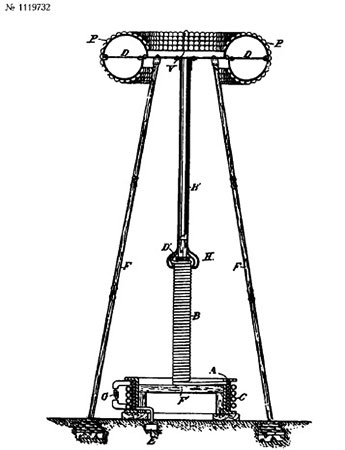In principle, without considering specific technical peculiarities, Tesla Tower is helically formed quarter-wave resonator grounded by one tail with distributed parameters, and additional capacity being located in the upper end of the helix.

This resonator is oscillated by means of reference generator (sinusoidal signal, oscillation frequency value is lower than 20 kHz if based on Tesla's patents such as US787412 and US1119732).

In other words, principal circuit of the tower looks as follows: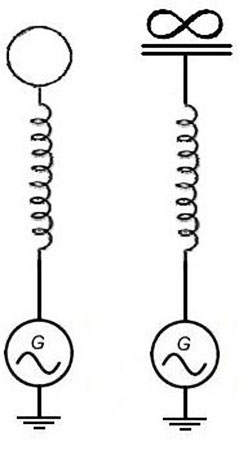On the left, you can see physical isolated capacity located in the top of tower (complements own coil's capacity).

On the right, you can observe conditional equivalent circuit, where it is especially outlined that the capacity is isolated. According to that drawing, capacity is located between Tower and perpetuity and not between Tower and the Earth as otherwise we would get conventional LC-chain grounded through the Earth).

For the purpose of minimization of stray capacity between the Tower and the Earth, that is, shortcutting LC-chain of the Tower through the Earth, it is necessary to elevate isolated capacity from the ground. Simple assessment demonstrated that it is needed to elevate the capacity to the height, which is equal on its value to several average diameters of such capacity.

At the observation of such condition, capacity between the Tower and the Earth will decrease on its value up to the value, which is comparable with own isolated capacity of the Tower.

It is well known from basics of classic electrical engineering that capacitive and inductive impedance cancel each other in a resonance mode.

Therefore, generator "observes" only active resistance of the resonator. Standing wave with the voltage node in the generator point occurs in the helix. Current loop is located there, as well (at that, voltage loop and current node are located in the resonator's tail).

More detailed analytical theory of the working principle of such resonator can be found here.

If material located there is too complex to be understood, it is possible to express everything said above in the following way: helically formed resonator of such kind simply represents quarter-wavelength folded up into helix. That is, standing wave of the current-voltages will exist in such "stretched" transmission line in such resonator at the resonant frequency with the voltage node at the one end of a line and current node on its other end.

Essential different of that from the "stretched" long transmission line is only in amplified inductive and capacitive connection between neighboring segments of such a line due to the their geometrical proximity in helical configuration.

The latter  slightly alters resonant frequency and wave propagation speed along the line.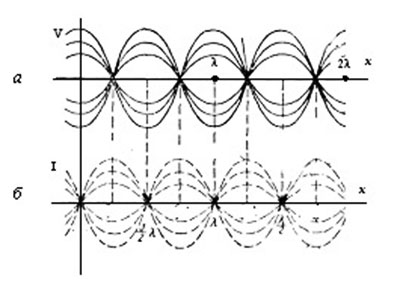In this Figure, you can see standing waves in long transmissionline.

Waves' distribution is as follows:

а - of the voltage;

b - that of the current in single-wired transmission line

(figure is taken from the following )

In other words, the Tower represents current buffer - isolated capacity inside of which reference power generator "brings" charge from the ground.

At that, EM-radiation in terms of radio waves (that is a field in distantly located wave zone of the Tower) is practically absent with regard to our range of working parameters. Let us demonstrate this in details.

There exists such a term as helical antennas in a field of radio physics. Primarily, such term can be related to such helix resonator. However, compared to antennas, electrical length of one turn of the Tower is smaller than wavelength by 1000 to 10 000 times (that is, number of turns can reach up to thousands considering the fact that total length of the coil is approximately equal to the quarter of wavelength).

At that, the greatest part of the currents (current loop) is concentrated in the lower half of the Tower. Hence, such a structure acts as classical conventional concentrated inductivity in terms of external EM-radiation. It is conventional magnetic dipole.

of the electrically short magnetic frame (magnetic dipole) with the wavelength denoted as λ is well known ( describes the losses of conductor for the radiation of EM-waves, i.e. current power loss for the radiation is considered as formal active resistance losses of which are equal to those spent for the radiation):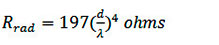(equation 8.148 can be found by checking the )

...where d is a diameter of magnetic dipole.

As of the case of N turns, the equation should be multiplied by the  factor denoted as N
2 (due to the radiation power density being proportional to the quadrate of the amplitude of a frame's field, i.e. quadrate of the number of the turns in the frame).

Let us make the following conclusion.Using our parameters such as frequency with the value of 10 kHz,i.e. wavelength of 30 000 m, coil radius being about 2 m, winding length being of 10km, and number of the turns being of 800, we will get the radiation resistance with the value of 0.04 micro ohms.

The latter is insignificantly small in comparison to the losses on the active resistance of a system, which comprises at least 1 ohm.

However, except of tangential constituent of the current in such resonator, there is also axial component (resulting vertical current) because of which the Tower provides radiation of the short electrical dipole for which the radiation resistance is interrelated with the length of dipole denoted as L and wavelength denoted as λ as follows: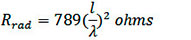(equation 8.81a can be found by checking the link indicated above)

The latter results in the value of about 0.0008 ohm in terms of our parameters and tower's height being about 30m, which is also insignificantly small in comparison to the losses on the active resistance of a system.

As a result, we can see that both types of radiation emitted from tangential as well as axial constituents of the current are insignificantly small with regard to the losses in active resistances of the Tower's helix considering that those are upper assessments (as for them, the current value is considered to be constant along all coil turns while in reality the current falls on the sine and there is current node or so-called current zero located at the "hot end" of the coil and real radiation value will be smaller by many times than that of the upper assessments).

So any kind of ideas of the Tower operating as an antenna is absurd (at least, while we adhere the Tesla's patents and do not exhibit any personal speculations).

The Tower cannot be considered as antenna in its classical understanding. Its radiation, that is, EM-field in the distant wave zone is insignificantly small and the tower can serve only as effective accumulator for charge, which is being brought in and out the ground by the generator at its working frequency.

Therefore, "genial" objections like "you will get conventional helical antenna with very low power transmission efficiency value" as well as any other "objections" based on radiation of such a structure only demonstrate total misunderstanding of the basic principles of radio physics by the opponent.

Now let us proceed with the Earth

To make it simple, let us start from simple analogies and then proceed with the final concept.

Let us assume  that we have electrically long conductor with the break in one tail and grounded by the other tail through the ac voltage source (and by electrically long we mean that the length of the conductor is comparable to/greater than that of the wave initiated by the generator based on the generator's frequency and wave propagation velocity being close to the light speed in vacuum):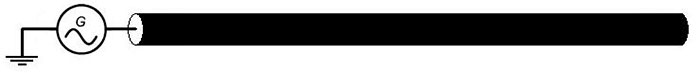In such long transmission line and in a case when losses within the line are very small, standing wave of the currents and voltages occurs (i.e. superposition of the incident waves from the generator and the waves reflected from the free end of the long line).

Conventional electrical vibrator or classical antenna can be considered as a typical example of such lines and waves as shown in the Figure below.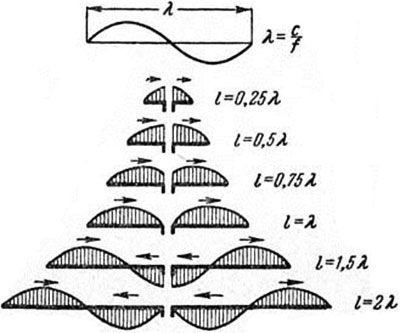Current distribution in symmetrical vibrating-reed relays of different length

It is very easy to understand the functionality of standing waves in the long transmission line.

Let us assume that conductor is divided into the segments with the length being half-size of that of the wave. Each of such segments is considered as a capacity (as conductor has a capacity distributed along) and as an inductivity (in an analogous manner). Hence, standing waves are nothing else but current waves charging/discharging such the capacities.

That is, energy in such standing wave is saved as a charge and current in an interchangeable manner. Such a charge is either allocated along the conductor (along the sine wave) with the current values being equal to zero or in the form of the currents redistributed along the conductor (along the sine wave, correspondingly).

At this moment, surface charge density along the conductor is equal to zero. The latter just resembles the working pattern of conventional LC-chain (induced coil sequentially connected with the condenser capacity) but with regard to distributed manner of capacity and inductivity. The currents in half-wave are "drawn" to the center of such selected segment creating voltage loop i.e. occurrence of surface charge in the conductor.

As of the neighboring segment, such currents are "drawn away" from similar center creating a charge of opposite sign.

This process is repeated further to the opposite side whilst creating the charges of opposite sign at the surface of a conductor. It is obvious that all aforesaid implies ideal transmission line without losses and being open-ended at the tail. More complex processes are happening in real transmission line with the losses and/or line with the load at the tail.

However, it does not affect principal essence of the subject in question.

As of elementary mechanical parities, the closest process will be one with the dilatational waves in the long spring, which do occur in a case when such spring laying at the support with zero friction is swayed back and forth along the axis of spring located in one end of the spring with the other end being fastened.

At that, movement velocity of the respective part of a spring corresponds to the current value, and an extent of spring compression depends on the voltage value. Thus, all parts of a spring will acquire zero velocity at some point while extent of spring compression will vary on sine and along spring so it may resemble some kind of alternative nodes and discharges.

The latter is determined by zero current in standing wave and maximum voltage value simultaneously (i.e. maximum value of surface charge density in the conductor).

It is also possible that whole spring will not be deformed over quarter of period of time, however actual velocity of its parts will vary on sinus along axis of a spring at the moment (the moment of zero charge density but with a maximum current value along the long line conductor correspond to that).

In general, losses in such transmission line can be divided in two constituents: ohmic losses and losses by radiation.

In a case of conductor having great length and small ohmic resistance, radiation will significantly affect the losses (i.e. radiation resistance). It is well know that if such a line will be surrounded by grounded conductive screen then losses by radiation will be neutralized.

Such structure is called as coaxial waveguide. It is noteworthy that in our example, wave in such coaxial waveguide will exist in a form of ТЕМ-mode (generator connected via ground to the internal and external conductors of a waveguide will serve as an excitation port).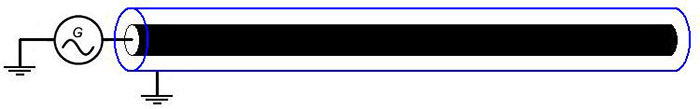Actually, state of ТЕМ mode can be considered as a state of inductive connection of internal and external conductors of waveguide via induction field in these conductors (current change in the internal core evokes EMF in the external screen. At that, induced current in the external screen directed against the current change in the internal core, i.e. it is actually conventional induction in the near field of a current).

Therefore, cross flows of energy are not only of a zero value for both ТЕ and ТМ mode over time in average, but they are also of a zero value at any moment of time. Re-reflection from the boundaries of waveguide does not take place.

In this case, energy flow has dilatational nature, that is, directed along the axis, and correspondingly Poynting's vector is directed also in strictly parallel direction of wave propagation along the axis of such coaxial resonator.

Therefore, state of
ТЕМ mode in coaxial waveguide is characterized by fine parameters concerning states of ТЕ and ТМ mode, in particular with regard to energy transmission and small value of wave attenuation coefficient in waveguide. Therefore, it is quite common to use state of TEM-mode when it is necessary to transmit energy via coaxial waveguide.

However, even in case of removal of grounding of the external screen of such waveguide, the screen is still finely capable of operating along entire length except of his end parts.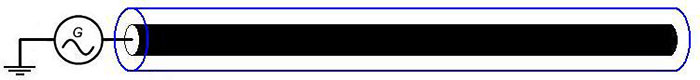This is due the fact that such screen represents transmission line anyway, where EMF of alternating current in internal conductor-core is used as a generator.

And some kind of voltage loop will exist only in the end parts of such screen due to small value of capacity of these ends. The rest part of screen will be fully functional. The latter can be proven by means of elementary modeling in Ansoft HFSS software for example.

The next question is what is going to happen if we will not remove grounding of external screen but will "close" the ends like it is demonstrated in Figure below (in this case, external screen will resemble some sort of "capsule"). The answer is quite clear - this situation will be absolutely similar to that described above.

Screen will operate on its entire length but the endings of such "capsule" will gather voltage loops as well as current nodes.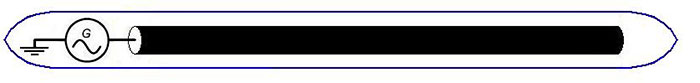Even if internal and external conductors can be designed as the spheres, we will end up with the general model of suggested experiment (proportions in Figure below were not adhered):It is easy to guess that internal conductive sphere is the Earth while upper layers of atmosphere (mainly ionosphere) represent internal conductive sphere.

And general geometry of such resonator is just conventional concentric spherical resonator in which only ТЕ and ТМ mode exist so we can't talk only of TEM mode. However, such resonator has quite unconventional way of excitation of the ТМ-mode (that is, excitation port does not connect external and internal plates like it is accomplished in "classical" electrical engineering).

Due to variable section of internal and external conductors, amplitudes of standing waves of the currents and voltages will decrease with distance from the generator. In this case, general essence of  this situation remains similar -
ТЕM mode of coaxial or ТМ-mode of spherical resonator
is excited by corresponding source (Tesla Tower).

First, this kind of idea seems strange - it is well known that conductivity of the Earth ground as well as that of ionosphere in clear day on the illuminated side is about 0.001 Cm/m (±period) while conductivity of copper is 58 000 000 Cm/m. However, let consider this issue based on computational assessments and not on intuitive reflections. Let us figure out the issue with the resistance of the Earth ground.

We assume that from the viewpoint of current flow processes, division to dielectrics, semiconductors and conductors is quite conditional on its nature as dielectric becomes comparably good conductor at its great section (that is, dielectric has small total resistance value while has small conductivity also).

It is well known that at sufficient thickness of conductor, current has substantial value only at some depth called as depth of skin-layer, which is calculated according to the
next equation:...where,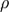- electrical resistance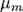- relative magnetic conductivity- frequency

Obviously, this is a simplified equation, which can be used for the conductor but not for dielectric. However, the losses related to permittivity of the grounds are very small at our ultra low frequencies.

Therefore, this equation can be used for performing an assessment.

As of the frequency range of 1-10 kHz and range of conductivities being of 0.001-0.00001 Cm/m, the depth of skin-layer lies within a range varying from hundred of meters up to several kilometers. At that, the lower will be the frequency the greater will be the depth of skin-layer, that is, the smaller will be ohmic losses in planetary resonance (inversely proportional to the root from frequency).

Thus, we can conclude that it is necessary to confine ourselves to the kilometer long layer considering active resistance of the Earth as a ball made of ground, that is, material with conductivity being at the level of 0.01-0.0001 Cm/m) and assuming that frequency range is not lower than 1 kHz (due to the fact that frequency with smaller values cannot be created from practical viewpoint based upon required technical parameters of the Tesla Tower).

Let us notice that obviously Tesla sincerely assumed that currents from his tower are directed inside of the Earth and not to its surface as it is indicated in our scientific article. According conventional electrodynamics, this is wrong assumption.

Resistance between two bars absorbed into medium with bad conductivity such as ground is defined by
the following equation: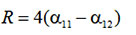Where,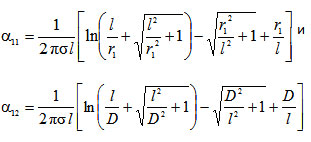L - length of the bars

D - distance between them

r1 - section radius of the bars- electric conductivity of the medium

It is noteworthy that based on this equation and starting from the distance between the bars, which exceeds the length of the bars, resistance between bars actually becomes constant (stops to increase as the distance grows).

For instance, typical resistance will be in a range of 1-3 ohm for two bars with the length of 30m and diameter of 0.2m as well as ground conductivity being about 0.04 Cm/m (which is correct for the upper layers of ground) starting from a distance of several meters and so forth (without restriction of remoteness of a distance) and at subsequent increase between bars.

Thus, it is intuitive delusion that the Earth is bad conductor (as an object in general). In opposite case grounding wouldn't make any sense - while it is used everywhere.

Peculiar feature of such equation is the fact that starting from some length of the bars further increase of the bar's length does not result in noticeable decrease of resistance between bars. In other words, cumulative resistance between receiver and transmitter insignificantly depends on the depth of skin-layer.

The latter is well-known fact in terms of grounding systems: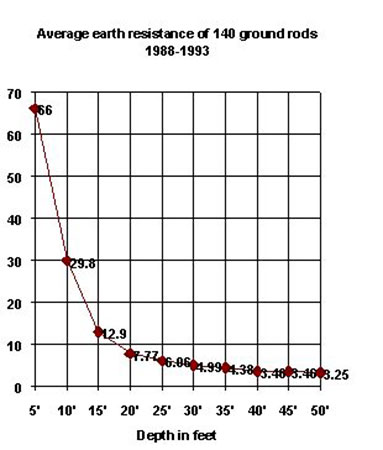Thus, we have to be optimistic in relation to resistance of entire surface of the Earth.

Let us perform an assessment in a more precise manner. Attenuation constant defining losses on the walls of the waveguide due to active resistance for ТЕМ-mode of coaxial waveguide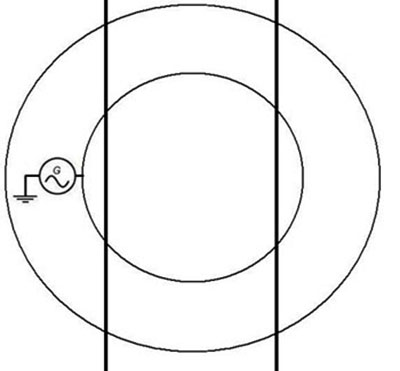(to which the biggest central part of the Earth-resonator is most closely located as shown in Figure above) is calculated according to the following equation (see for example here or - eq. 10.4.8):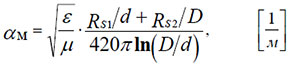...where Rs1 and Rs2 are surface resistances of metal of internal and external cylinders of the waveguide, which can be calculated according to the following equation: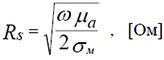Here µ is (for the most parts of superficial ground it is just ).

Let us notice that there is ratio of frequency and conductivity located under root, that is, conductivity which is smallest on its value compared to that of the metals is mostly compensated by kHz-frequency range (while coaxial waveguides are used for GHz-frequency range).

The fact of their ratio being located under root sign "improves" situation even more.

Thus, we receive variable with the value of 1.06 ohm for our parameters such as f=3 kHz and σ=0.01 Cm/m which is typical value of surface resistance of both The Earth and ionosphere with the 1ohm-period±period.

1ohm seems to be still quite large number. However, cavity Q factor is proportional to its linear dimensions due to the fact that energy amount in resonator is proportional to its volume and losses are proportional to the area of the resonator's walls. The latter is reflected in the equation, in numerator.

In our case, radiuses D and d have great value (D=6.600.000 m, d = 6.400.000 m), which completely exceeds relatively great value of surface conductivity of the walls of the waveguide.

Therefore, attenuation constant for our parameters can be assessed using the equations indicated above as having value of 10-8-10-9 1/m.

In reality, the biggest part of the planet surface is covered by the fine electrolyte (salty oceanic water), i.e. given evaluation is upper bound while real attenuation constant will be lower.

Attenuation constant equal to 10
-9 means that wave amplitude will decrease by 1-e-x10^(-9)=2% along all the length of way "x" of wave from opposite point of globe being about 20000km.

Extremely high Q-factor of resonator Earth-Ionosphere corresponds to that phenomenon described above (its value is much greater than one hundred) for such mode in comparison to mechanism of distribution of conventional radio waves via re-reflection from Earth-Ionosphere borders. And even worsening of assessed conductivity by 10-100 times (which is sensible in terms of ionosphere) does not result in fatal consequences in terms of the fact that such resonance might exist.

We came to conclusion that, in principle, sought resonance can take a place based on physical parameters of a resonator, however real Q-factor of such resonance can have plug by 10-100 times (but even at the worst combination of parameters its value shouldn't be lower than one hundred).

Similar assessment of possible high Q-factor of
ТМ-modes in the resonator Earth-Ionosphere was demonstrated in  and it was referred as "modes of multilayered concentric spherical resonator".

As of rather strict approach, it is necessary to consider normal concentric resonator in
ТМ-mode state (for instance, decent review on this question can be found at , otherwise individuals interest in more deep theoretical aspects can check or works).

First harmonics of zero
ТМ-mode correspond to the phenomenon of so-called Shuman resonance.

However, speaking of frequencies with the value range being within few kHz, except of zero mode the following ones will be subjected to excitation (for 10 кHz these are mode numbers within range of 0-6).

Hence, as it can be seen from equation,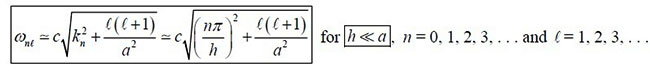...for the first mode - the lowest harmonic will have frequency with the value of about 1.5 kHz, and for the second mode this value will be 3 kHz etc.

At that, as it can be seen from the equation defining harmonic frequencies for each of such modes starting from the first one and so forth, "density" of harmonics location along frequency axis is quite big (for instance, if harmonics come with an interval of about 10Hz for zero mode then for the rest of modes within frequency range being below 10kHz this value will be with an interval of about 0.01-0.1Hz).

Therefore, it is impossible to talk about any concrete mode/harmonic while performing excitation of ТМ-modes of such resonator at the frequency range being of few kHz: final situation with standing waves will correspond to exceedingly large amount of harmonics for several modes at once.

The latter serves as a principal difference of such resonance from Shuman's one.

There is also another principal difference. It is well known that (
see here - p. 8) for passive resonator, Q-factor of harmonics increases with frequency growth in a manner, which is approximately proportional to its root.

However, resonator Earth-Ionosphere is not passive on its nature. In reality, electrical machine of the Earth keeps approximately constant voltage difference between the plates of planetary condenser (ground-atmosphere). In case of strike of lighting, given voltage decreases, however its value returns to normal during definite period of time measured in seconds.

At that, typical charging current density comprises about 0.1-1A per square kilometer. In other words, the Earth operates as EDS source, which slowly stabilizes voltage difference at some kind of average level. Obviously in case of ultra-low frequency oscillations corresponding to the Shuman's resonance (first harmonics of zero ТМ-mode of resonator), presence of such EDS source results in significant worsening of Q-factor of resonance.

In case of voltage potential fluctuation from  average level, this EDS source aims at compensating of the fluctuation.

The latter implies active suppression of ТМ-mode which can have significant effect taking into consideration planetary scale of this phenomenon. Unfortunately, this factor is not considered in any of known models of TM-mode resonance in Earth-Ionosphere resonator.

The reasons for that are quite understandable - there is no any definite model of mechanism of occurrence of such EDS source as well as this mechanism is significantly non-linear in terms of any kind of phenomenon related to atmospheric electricity. Therefore, any kind of adequate modeling (consideration) of such factor for ТМ-modes of resonator is not possible due to lack of the data.

Nevertheless, the data are known concerning Q-factor of the first harmonics of zeroТМ-mode of the resonator Earth-ionosphere (these data were collected in 2011):

 Harmonic frequency f, Hz 7.8 14.3 20.8 27.3 33.8 Q-factor 3.5 4.5 6.2 7.7 8.2 Simple extrapolation from the first harmonic (for the passive resonator Q-factor value is proportional to the radical from the frequency value) 4.7 5.7 6.5 7.3

As it can be seen from this table, actual Q-factor value increases quickly with the subsequent increase of a number of the harmonics compared to the radical from the frequency value (i.e. it increases faster than for passive resonator).

This mechanism is going to have the greatest influence taking into consideration relative "slowness" of charging mechanism of planetary condenser exactly for the lowest zero mode harmonics due to the fact that Q-factor value of resonance will increase with quite great speed along with subsequent increase of the frequency value.

It is noteworthy that in 2011 (the latter is reflected in the link provided above), the currents were discovered in the ionosphere, which were corresponding to Shuman's resonance at the altitude of 400-800 km for which all prior models were not providing any kind of the currents at all. In fact, existing models of ionosphere conductivity appeared to be improper and useless in terms of elaboration of models of resonance of
ТМ-mode in the resonator Earth-Ionosphere.

Thus, there are grounds existing for conducting direct measurements of Q-factor value of total resonance at the frequencies with the value being of only few kHz. This can be accomplished because of theoretical references and actual results obtained by Tesla. Direct analytical or computational calculation is impossible to perform due to complex nature of not so well known object Earth with great amount of own parameters/features.

Actually, suggested experimental concept is neither related to Shuman's resonance (and other Q-factors as well as additional
ТМ
-modes and resonance harmonics) nor to energy transmission via radio waves of EM-field of distance wave zone of conventional radiation units. And Tesla was directly stating that.

Like it was said above, as resonator's section is not constant then wave impedance (defining voltage ratio to the current in the line) will vary: maximum voltage will take a place in the loops located in direct proximity to the tower (and from opposite end of the planet), minimum at the "equator" of the Tower.

The latter can be confirmed by computational calculations performed by means of Ansoft HFSS software and using corresponding  like, for instance, for zeroТМ-mode of such resonator: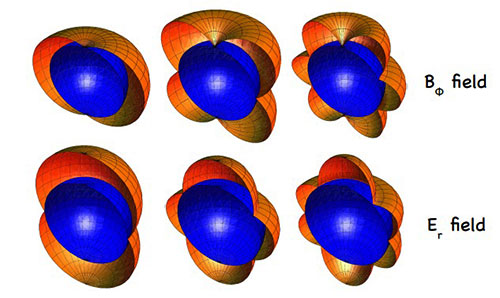Distribution of the amplitudes of electrical field E and magnetic field B for the first 3 harmonics of zero ТМ-mode of resonator Earth-Ionosphere, the Figure was taken from here.

As is known in theoretical physics, there is so-called , defining the distribution of EM-field over the surface of the medium with finite conductivity (in the case of an arbitrary location of the radiation source on the boundary between two media).

We could try to use this expression to create the analytic theory of how Tesla Tower works. Indeed, the tower itself can be seen as a set of EM-sources distributed along the height of the Tower, and then using the Sommerfeld integral, we may try directly derive an analytical expression for the wave of current-voltage induced in the ground by the Tesla Tower.

Alas, although itself Sommerfeld integral has been known about 100 years, for all these 100 years, despite the efforts of prominent theorists in the field of physics and mathematics, no one was able to find an analytical solution for the Sommerfeld integral.

It would be naive to believe that we are able to solve this theoretical problem which was not solved by the best minds of the world over 100 years... Thus, all that remains for us - is to be satisfied with the numerical calculation only, which we did - using Ansoft HFSS (as discussed below).

It is noticeable that accordingly Sommerfeld integral, a so-called surface wave (or ground wave) may be generated in case of dipole source placed on the boundary between ground and air. In general Tesla tower is similar to that source. So accordingly Maxwell equations surface wave has to exist at least at short distance (several wavelengths) near the tower.

Numerical calculation on HFSS software do confirms that such surface wave can be easily generated by Tesla tower as it is shown at the picture below (Earth is placed in infinite space and has no atmosphere in this calculation - so there is no reflection from ionosphere, nevertheless surface wave still exists; Tower is placed near the right bottom corner).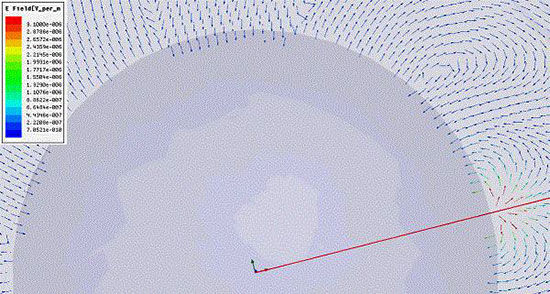"Grounding" for the resonator Earth-Ionosphere

The question is where from we are going to get "grounding" to which generator for pumping such resonator is connected as it is shown in the figure below?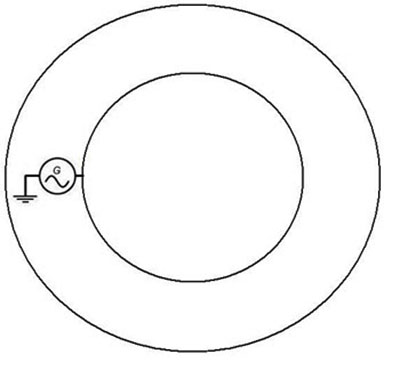The answer is simple as we have already examined working principle of the Tesla Tower.

From the viewpoint of generator, Tesla Tower is not any different from some kind of external ground (connected via active resistance of the Tower). This is due to the fact that generator "sees" only active resistance of the Tower but does not react anyhow at the charge value, which is accumulated in the tower.

This is because of capacitive and inductive resistance in the resonance mode compensate each other. In other words, for generator the Tower is some sort of "grounding" via resistance which is equal to active resistance of the Tower.

As it was noticed above, such charge evokes distribution of the charges in ground located in direct proximity next to the Tower. However, the higher charge integrator of the Tower is elevated the less important is that factor as capacity Earth-Ground is decreasing.

It is enough to elevate charge integrator to the height which is significantly higher that the size of integrator so that the Tesla Tower would really start to serve as "external ground" for the reference generator (i.e. it is enough to minimize the capacity between the Tower and Earth so  that own isolated capacity value of the Tower would become at least somewhat equal to that of the capacity Tower-Earth).

Energy transmission efficiency coefficient

Upon establishment of the standing waves of the voltages and currents over all the planet (at that, currents will have quite small amplitude in comparison to the voltages) it is possible to conduct efficient removal of this energy by the analogous system (the same Tower but without generator).

Physical process taking place during the work of a receiver are characterized by the establishment of the connection between Tower-Receiver resonance circuit. The latter allows obtaining quite high transmission efficiency coefficient even at the low coupling coefficient of the source and receiver strictly in correspondence with conventional  electrical engineering.

Let us proceed with analysis of this issue in more detailed manner.

In a case of receiver, i.e. similar circuit being located in voltage loop and the current node of the final standing wave, alternating potential of the surface will serve as EDS for receiver. At that, there will happen resonance excitation in the receiver in completely similar manner as that in the source.

Correspondingly, receiver will generate ground wave in a manner similar to that of the source. In addition, as receiver is located in voltage loop and the current node, the generated wave will create additional load to the source and, thus, form the system in a shape of so-called resonance coupled circuits (quick review on this question can be found here and here).

In fact, receiver has voltage node in voltage and current loops of external wave as well as operated at the same frequency, that is, in a field of location of the source where the receiver will create voltage loop and current node and which the source will "see" as additional load.

It can be very well observed in the videowhich illustrated basics of computational modeling by means of Ansoft HFSS software.The source is located in the left upper field in the video and in the Figure above, location field of the receiver is selected in the right near-to-central part.

It can be seen that the  minimum value of a field in a field of the receiver is constant. The latter indicated efficient removal of the energy from the planetary resonance. Interference image of the waves emitted by the receiver and source is also very clearly visible.

For such kind of systems, that is, resonant coupled circuits, energy transmission efficiency coefficient is determined by multiplication of system coupling coefficient k and its Q-factor. Coupling coefficient defines what part of energy of circuit-source resonance is "seen" by the circuit-receiver.

For instance, coupling coefficient is reaching 1 for the closely located inductive coils (especially if they are spooled on same iron) and subsequently decreases as coils get separated due to the EDC decrease.

Typical graph of efficiency factor's dependency η from intersection of the coupling coefficient k to Q-factor is provided below: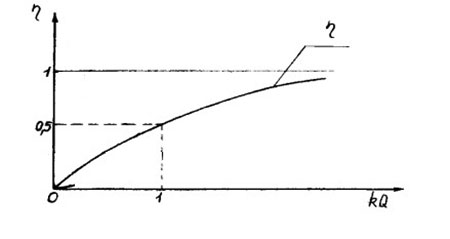Physical meaning of such dependency is obvious - even in case when receiver "takes" only small percent of source energy during one oscillation period, energy transmission efficiency factor determining relation of transmitted and dissipated energies will have great value due to the energy losses being small in total resonance during same period of time because of high Q-factor of resonance.

That is, high circuit coupling coefficient is not required for achieving high energy transmission efficiency coefficient value. This is because Q-factor with great value can compensate small value of the coupling coefficient.

Let us assess coupling coefficient between source and receiver and, supposedly, Q-factor of resonator Earth-ionosphere with high value (we have all needed grounds for this assumption based on the facts discussed above).

Let the frequency have a value of 10kHz. It means that the Earth is divided to "rings" with the width being of 30km from which around 700 is accounted for the length of half of perimeter. The capacity of the Earth as isolated conductor has a value of about 700 μF.

Let the current in the Tower (source) have a value of 1kA, which corresponds to the power of generator being at least few MW.

As of the long transmission line - the Earth, our "rings" serve as parallel capacities. That is, capacity per one wavelength within the "Equator" next to the Tower can have a value of 1 μF (700μF/700 waves). The latter provides the voltage with the value of 15kV according to the standard equation such as: U=I*Rc=I/(c1*w) and at the current value being of 1kA (directed for charging of such capacities.

Entire field for TM-mode is concentrated on the length which is equal to the half of wave that is vertical to the ground as it can be seen from computational modeling performed by means of HFSS (and/or corresponding analytical equations links to which were provided above).

For 10 kHz it is 15km.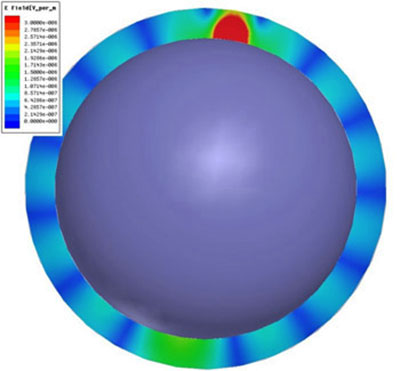Field gradient has a value of only one volt pet meter (at the background gradient of vertical constituent of the field being around.

Such value is maintained in the "Equator" and what comes to the loops located closely to the Tower the capacity value will be by 1 or even 2 times greater. That is, the Tower-receiver will "notice" voltage value of 100kV (and in such case field gradient will have value of 10V/m) in case when located at the distance of dozens of kilometers from the source.

In this situation, alternating potential of the ground has very big value and field gradient value is so small due to field being distributed vertically along the great distance of dozens of kilometers. The latter allows speaking of even greater power values of a receiver in order do not get away from the gradient level of field located next to the Earth.

In case of the "Equator" and at indicated parameters and total resonance voltage in source having MV value and in the equator having that of 10kV, coupling coefficient will be of 1%, correspondingly (and dozens of % at the distance of dozens of kilometers from source).

This is because of coupling coefficient can be defined as ratio of voltages at the receiver's inductivity at opened circuit of a receiver to the working source given the same parameters of receiver and source. Based on probable Q-factor of resonance being with the value of few hundreds, such coupling coefficient implies energy transmission efficiency ratio being with the value of at least dozens of % for the equator and even can be with a value of above 90% for the distance accounting dozens of kilometers.

The latter completely corroborates with the statements made by Tesla after conduction of corresponding experiments.

So, in general, it is possible to expect that two Tesla towers (transmitter and receiver) forms capacitive coupled resonant circuits, with capacitive coupling caused by surface waves (in case of  short distance between towers, up to several hundreds of kilometers) or waves in Earth-Ionosphere waveguide (for long distance between towers) generated by both towers.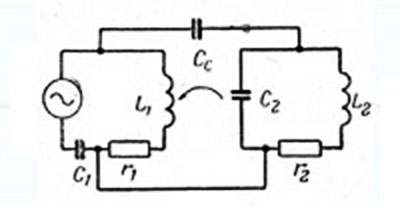Lumped-circuit scheme

of resonant coupling of Tesla Tower

However, due to problematic issues related to computational modeling and calculations of actual Q-factor of resonance it is not reasonable to attempt to make more precise evaluation (actually all depends on actual Q-factor of the resonator-Earth and resonator-Tower since modeling can result in significant errors).

So the only adequate way of conducting assessment would be elaboration and carrying out full scale experiment. Obviously, for that purpose it is necessary to design full analogue of the Tesla tower.

The latter will allow real representation of the actual "Tesla Tower" and conduction of actual "correct experiment" and subsequent clarification of issue concerning energy transmission efficiency coefficient in terms of energy transmission over the long distances.

At the same time, we have no doubts that the energy transmission efficiency coefficient will have a great value for experimental setup similar to original parameters (taking place in the Tesla's experiments, i.e. in terms of distances ranging at least within hundreds of kilometers).

The latter is interesting from practical viewpoint in any case.

In addition to the patents related to the Tower, Tesla also patented device for location of standing waves of voltages in the ground occurring as a result of lighting strike.

This device is described in patent US787412. The main working principle of given detector is based on ensuring the operating process of so-called synchronous detector or ).

Some Wikipedia statements are provided below (and are wrong):

The lock-in amplifier is commonly believed to be invented by Princeton University physicist Robert H. Dicke who founded the company Princeton Applied Research (PAR) to market the product.

However, in an interview with Martin Harwit, Dicke claims that even though he is often credited with the invention of the device, he believes he read about it in a review of scientific equipment written by Walter C. Michels, a professor at Bryn Mawr College.

This was probably a 1941 paper by Michels and Curtis, which in turn cites a 1934 paper by C.R. Cosens.

It is obvious that Tesla's fellows did not sufficiently research other Tesla's ideas and patents on which he objectively had priorities.

However, more thorough analysis of device detecting standing waves and used by Tesla does not leave any doubts in the fact that invention of synchronous detector has been done by Tesla around 1900 year (not by Robert H. Dicke several dozens of years later).

In fact, Tesla used such device for the purpose of sequential closure of one of the contact of condenser-accumulator with the ground while other contact was "in the air" at the given frequency and pulse ratio (see patent) by means of purely mechanical approach using sliding contacts in corresponding cylinder denoted as F in the Figure below.Thus, given the condition of closing frequency coalescence of standing wave in the ground and closing frequency of contacts in receiver, condenser Т was gradually accumulating the charge and then involuntary was discharged via receiver R allowing registration of discharging current of such accumulating condenser.

The latter reflects typical logics of lock-in amplifier. At that, as the length of cables connecting condenser with ground was much smaller than wavelength it is not wise to talk of EM-crosstalks for such cables (from lighting strikes) - they are going to be too small.

Here is something Tesla was writing about this and that is where from his path took a start in this field:

The date I shall never forget - when I obtained the first decisive experimental evidence of a truth of overwhelming importance for the advancement of humanity.

A dense mass of strongly charged clouds gathered in the west and towards the evening a violent storm broke loose which, after spending its fury in the mountains, was driven away with great velocity over the plains. Heavy and long persisting arcs formed almost in regular time intervals. My observations were now greatly facilitated and rendered more accurate by the experiences already gained. I was able to handle my instruments quickly and I was prepared.

The recording apparatus being properly adjusted, its indications became fainter and fainter with the increasing distance of the storm until they ceased altogether. I was watching in eager expectation. Surely enough, in a little while the indications again began, grew stronger and stronger and, after passing thru a maximum, gradually decreased and ceased once more.

Many times, in regularly recurring intervals, the same actions were repeated until the storm, which, as evident from simple computations, was moving with nearly constant speed, had retreated to a distance of about three hundred kilometres. Not did these strange actions stop then, but continued to manifest themselves with undiminished force.

Subsequently, similar observations were also made by my assistant, Mr. Fritz Lowenstein, and shortly afterwards several admirable opportunities presented themselves which brought out still more forcibly and unmistakably, the true nature of the wonderful phenomenon. No doubt whatever remained: I was observing stationary waves.

Based upon actual detector's design there are no any doubts in the following fact that evidence of operating process of such detector, and in particular, periodical sinusoidal change of amplitude of energetic of the process itself along progress and recession of storm to the hundreds of miles and being registered by the detector definitely implied the fact of presence of standing voltage waves in the ground of Earth.

The latter induced Tesla to commencing of his research.

Based on all information provided above we can assume that there are all necessary grounds for conduction of full scale experiment for the purpose of final confirmation of the important use of the Tesla Tower.